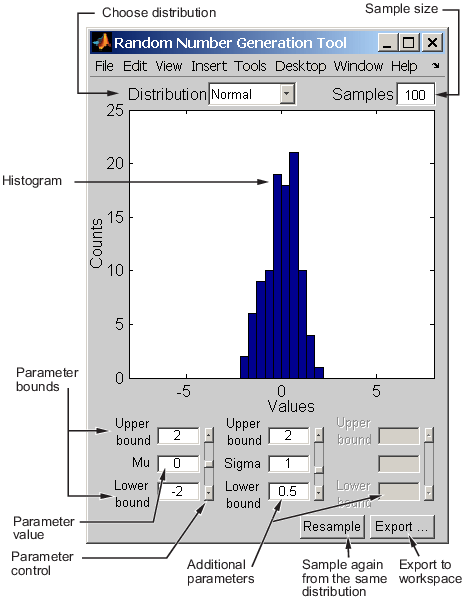Documentation

Working with Probability Distributions

Probability distributions are theoretical distributions based on assumptions about a source population. The distributions assign probability to the event that a random variable has a specific, discrete value, or falls within a specified range of continuous values.

Statistics and Machine Learning Toolbox™ offers several ways to work with probability distributions.

For a list of distributions supported by Statistics and Machine Learning Toolbox, see Supported Distributions.

You can define a probability object for a custom distribution and then use the Distribution Fitter app or probability object functions, such as pdf, cdf, icdf, and random, to evaluate the distribution, generate random numbers, and so on. For details, see Define Custom Distributions Using the Distribution Fitter App. You can also define a custom distribution using a function handle and use the mle function to find maximum likelihood estimates. For an example, see Fit Custom Distribution to Censored Data.

Probability Distribution Objects

Probability distribution objects allow you to fit a probability distribution to sample data, or define a distribution by specifying parameter values. You can then perform a variety of analyses on the distribution object.

Create Probability Distribution Objects

Estimate probability distribution parameters from sample data by fitting a probability distribution object to the data using fitdist. You can fit a single specified parametric or nonparametric distribution to the sample data. You can also fit multiple distributions of the same type to the sample data based on grouping variables. For most distributions, fitdist uses maximum likelihood estimation (MLE) to estimate the distribution parameters from the sample data. For more information and additional syntax options, see fitdist.

Alternatively, you can create a probability distribution object with specified parameter values using makedist.

Work with Probability Distribution Objects

Once you create a probability distribution object, you can use object functions to:

• Compute confidence intervals for the distribution parameters (paramci).

• Compute summary statistics, including mean (mean), median (median), interquartile range (iqr), variance (var), and standard deviation (std).

• Evaluate the probability density function (pdf).

• Evaluate the cumulative distribution function (cdf) or the inverse cumulative distribution function (icdf).

• Compute the negative loglikelihood (negloglik) and profile likelihood function (proflik) for the distribution.

• Generate random numbers from the distribution (random).

• Truncate the distribution to specified lower and upper limits (truncate).

Save a Probability Distribution Object

To save your probability distribution object to a .MAT file:

• In the toolbar, click . This option saves all of the variables in your workspace, including any probability distribution objects.

• In the workspace browser, right-click the probability distribution object and select Save as. This option saves only the selected probability distribution object, not the other variables in your workspace.

Alternatively, you can save a probability distribution object directly from the command line by using the save function. save enables you to choose a file name and specify the probability distribution object you want to save. If you do not specify an object (or other variable), MATLAB® saves all of the variables in your workspace, including any probability distribution objects, to the specified file name. For more information and additional syntax options, see save.

Analyze Distribution Using Probability Distribution Objects

This example shows how to use probability distribution objects to perform a multistep analysis on a fitted distribution.

The analysis illustrates how to:

• Fit a probability distribution to sample data that contains exam grades of 120 students by using fitdist.

• Compute the mean of the exam grades by using mean.

• Plot a histogram of the exam grade data, overlaid with a plot of the pdf of the fitted distribution, by using plot and pdf.

• Compute the boundary for the top 10 percent of student grades by using icdf.

• Save the fitted probability distribution object by using save.

Load the sample data.

The sample data contains a 120-by-5 matrix of exam grades. The exams are scored on a scale of 0 to 100.

Create a vector containing the first column of exam grade data.

Fit a normal distribution to the sample data by using fitdist to create a probability distribution object.

pd = fitdist(x,'Normal')
pd =
NormalDistribution

Normal distribution
mu = 75.0083   [73.4321, 76.5846]
sigma =  8.7202   [7.7391, 9.98843]

fitdist returns a probability distribution object, pd, of the type NormalDistribution. This object contains the estimated parameter values, mu and sigma, for the fitted normal distribution. The intervals next to the parameter estimates are the 95% confidence intervals for the distribution parameters.

Compute the mean of the students' exam grades using the fitted distribution object, pd.

m = mean(pd)
m = 75.0083

The mean of the exam grades is equal to the mu parameter estimated by fitdist.

Plot a histogram of the exam grades. Overlay a plot of the fitted pdf to visually compare the fitted normal distribution with the actual exam grades.

x_pdf = [1:0.1:100];
y = pdf(pd,x_pdf);

figure
histogram(x,'Normalization','pdf')
line(x_pdf,y)The pdf of the fitted distribution follows the same shape as the histogram of the exam grades.

Determine the boundary for the upper 10 percent of student exam grades by using the inverse cumulative distribution function (icdf). This boundary is equivalent to the value at which the cdf of the probability distribution is equal to 0.9. In other words, 90 percent of the exam grades are less than or equal to the boundary value.

A = icdf(pd,0.9)
A = 86.1837

Based on the fitted distribution, 10 percent of students received an exam grade greater than 86.1837. Equivalently, 90 percent of students received an exam grade less than or equal to 86.1837.

Save the fitted probability distribution, pd, as a file named myobject.mat.

save('myobject.mat','pd')

Probability Distribution Functions

You can also work with probability distributions using distribution-specific functions. These functions are useful for generating random numbers, computing summary statistics inside a loop or script, and passing a cdf or pdf as a function handle (MATLAB) to another function. You can also use these functions to perform computations on arrays of parameter values rather than a single set of parameters. For a list of supported probability distributions, see Supported Distributions.

Analyze Distribution Using Distribution-Specific Functions

This example shows how to use distribution-specific functions to perform a multistep analysis on a fitted distribution.

The analysis illustrates how to:

• Fit a probability distribution to sample data that contains exam grades of 120 students by using normfit.

• Plot a histogram of the exam grade data, overlaid with a plot of the pdf of the fitted distribution, by using plot and normpdf.

• Compute the boundary for the top 10 percent of student grades by using norminv.

• Save the estimated distribution parameters by using save.

You can perform the same analysis using a probability distribution object. See Analyze Distribution Using Probability Distribution Objects.

Load the sample data.

The sample data contains a 120-by-5 matrix of exam grades. The exams are scored on a scale of 0 to 100.

Create a vector containing the first column of exam grade data.

Fit a normal distribution to the sample data by using normfit.

[mu,sigma,muCI,sigmaCI] = normfit(x)
mu = 75.0083
sigma = 8.7202
muCI = 2×1

73.4321
76.5846

sigmaCI = 2×1

7.7391
9.9884

The normfit function returns the estimates of normal distribution parameters and the 95% confidence intervals for the parameter estimates.

Plot a histogram of the exam grades. Overlay a plot of the fitted pdf to visually compare the fitted normal distribution with the actual exam grades.

x_pdf = [1:0.1:100];
y = normpdf(x_pdf,mu,sigma);

figure
histogram(x,'Normalization','pdf')
line(x_pdf,y)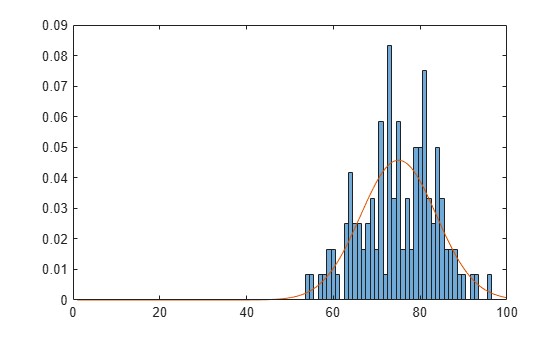The pdf of the fitted distribution follows the same shape as the histogram of the exam grades.

Determine the boundary for the upper 10 percent of student exam grades by using the normal inverse cumulative distribution function. This boundary is equivalent to the value at which the cdf of the probability distribution is equal to 0.9. In other words, 90 percent of the exam grades are less than or equal to the boundary value.

A = norminv(0.9,mu,sigma)
A = 86.1837

Based on the fitted distribution, 10 percent of students received an exam grade greater than 86.1837. Equivalently, 90 percent of students received an exam grade less than or equal to 86.1837.

Save the estimated distribution parameters as a file named myparameter.mat.

save('myparameter.mat','mu','sigma')

Use Probability Distribution Functions as Function Handle

This example shows how to use the probability distribution function normcdf as a function handle in the chi-square goodness of fit test (chi2gof).

This example tests the null hypothesis that the sample data contained in the input vector, x, comes from a normal distribution with parameters µ and σ equal to the mean (mean) and standard deviation (std) of the sample data, respectively.

rng('default') % For reproducibility
x = normrnd(50,5,100,1);
h = chi2gof(x,'cdf',{@normcdf,mean(x),std(x)})
h = 0

The returned result h = 0 indicates that chi2gof does not reject the null hypothesis at the default 5% significance level.

This next example illustrates how to use probability distribution functions as a function handle in the slice sampler (slicesample). The example uses normpdf to generate a random sample of 2,000 values from a standard normal distribution, and plots a histogram of the resulting values.

rng('default') % For reproducibility
x = slicesample(1,2000,'pdf',@normpdf,'thin',5,'burnin',1000);
histogram(x)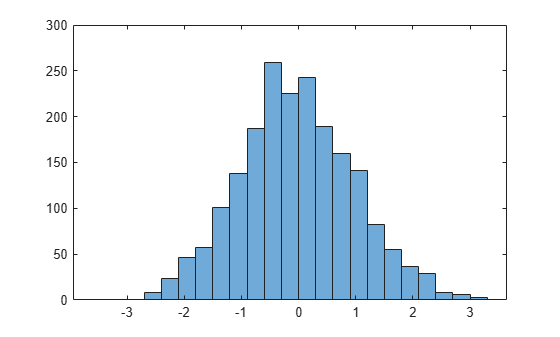The histogram shows that, when using normpdf, the resulting random sample has a standard normal distribution.

If you pass the probability distribution function for the exponential distribution pdf (exppdf) as a function handle instead of normpdf, then slicesample generates the 2,000 random samples from an exponential distribution with a default parameter value of µ equal to 1.

rng('default') % For reproducibility
x = slicesample(1,2000,'pdf',@exppdf,'thin',5,'burnin',1000);
histogram(x)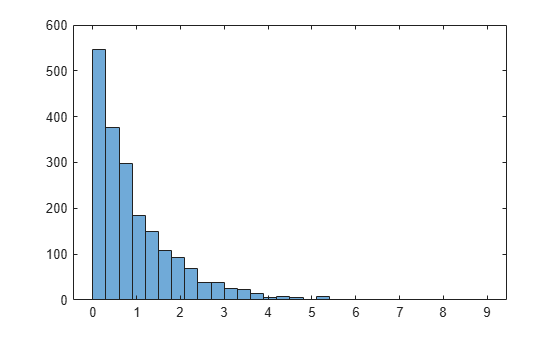The histogram shows that the resulting random sample when using exppdf has an exponential distribution.

Probability Distribution Apps and User Interfaces

Apps and user interfaces provide an interactive approach to working with parametric and nonparametric probability distributions.

Distribution Fitter App

The Distribution Fitter app allows you to interactively fit a probability distribution to your data. You can display different types of plots, compute confidence bounds, and evaluate the fit of the data. You can also exclude data from the fit. You can save the data, and export the fit to your workspace as a probability distribution object to perform further analysis.

Load the Distribution Fitter app from the Apps tab, or by entering distributionFitter in the command window. For more information, see Model Data Using the Distribution Fitter App.Probability Distribution Function Tool

The Probability Distribution Function user interface visually explores probability distributions. You can load the Probability Distribution Function user interface by entering disttool in the command window.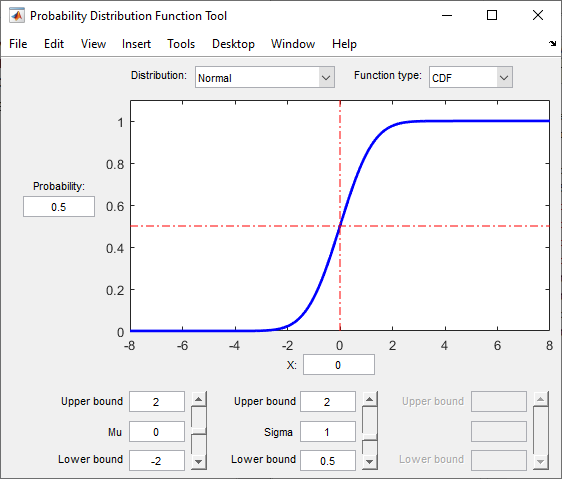Random Number Generation Tool

The Random Number Generation user interface generates random data from a specified distribution and exports the results to your workspace. You can use this tool to explore the effects of changing parameters and sample size on the distributions.

The Random Number Generation user interface allows you to set parameter values for the distribution and change their lower and upper bounds; draw another sample from the same distribution, using the same size and parameters; and export the current random sample to your workspace for use in further analysis. A dialog box enables you to provide a name for the sample.